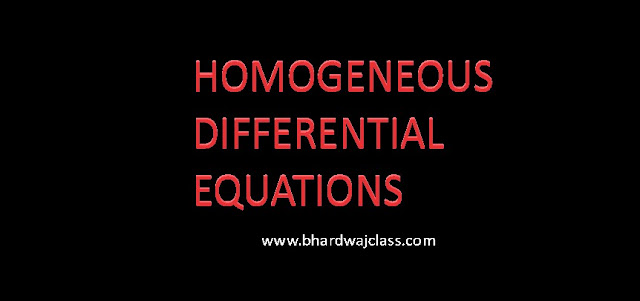# CBSE CLASS 12 DIFFERENTIAL EQUATION (HOMOGENEOUS) PRACTICE QUESTIONS

##HOMOGENEOUS DIFFERENTIAL EQUATION PRACTICE QUESTIONS

1.   Solve the differential equation $x 2 dy+y( x+y )dx=0$ given that y=1 when x=1.

2.   Solve the differential equation

3.   Solve

4.   Find the particular solution of the differential equation:

5.   Solve

6.   Solve:

7.   Solve the following initial value problem
(i)
(ii)  $2{x}^{2}\frac{dy}{dx}-2xy+{y}^{2}=0,\text{\hspace{0.17em}}y\left(e\right)=e$
(iii)  $\frac{dy}{dx}-\frac{y}{x}+\mathrm{cos}ec\frac{y}{x}=0,\text{\hspace{0.17em}}y\left(1\right)=0$
 (iv)

8.Find the particular solution of the differential equation

9.Find the particular solution of the differential equation

10.  Find the particular solution of the differential equation $\frac{dy}{dx}=\frac{xy}{{x}^{2}+{y}^{2}}\text{\hspace{0.17em}}given\text{\hspace{0.17em}}that\text{\hspace{0.17em}}y=1\text{\hspace{0.17em}}\text{w}hen\text{\hspace{0.17em}}x=0$